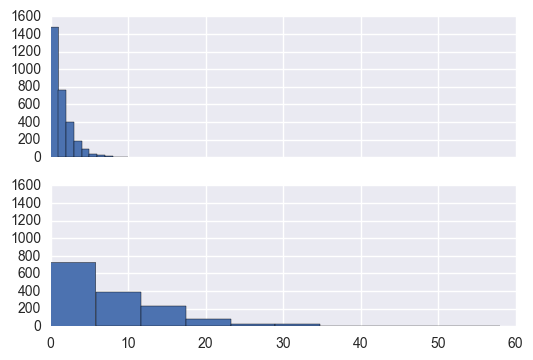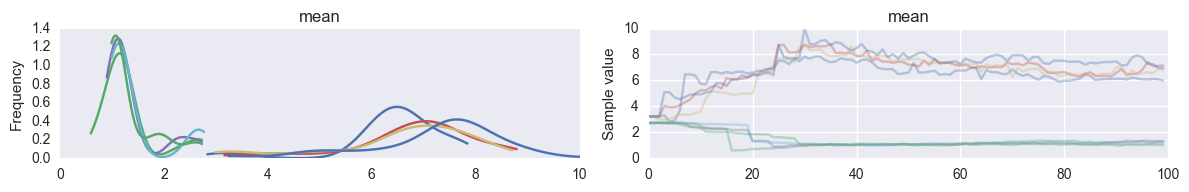In :
%matplotlib inline
import numpy as np
import statsmodels.api as sm
import pymc3 as pm
#import theano.tensor as tt
from theano import tensor as T
from matplotlib import pylab as plt
# import pystan
import seaborn as sns

np.random.seed(1)
n1 = 3000
n2 = 1500
n = n1 + n2

mu1 = 1
mu2 = 8

size = 1.2

data1 = np.random.negative_binomial(size, size/(mu1 + size), n1)
data2 = np.random.negative_binomial(size, size/(mu2 + size), n2)
data = np.concatenate([data1, data2])

In :
fig, axes = plt.subplots(2, 1, sharex=True, sharey=True)
axes.hist(data1)
axes.hist(data2);In :
with pm.Model() as model:

p = pm.Uniform( "p", 0 , 1)
ber = pm.Bernoulli( "ber", p = p, shape=len(data))

size = pm.HalfCauchy('size', beta=2.5)

mean = pm.Lognormal('mean', 1, 100, shape=2 )
mu = pm.Deterministic('mu', mean[ber])
process = pm.NegativeBinomial('obs', mu, alpha=size, observed=data)

with model:
trace = pm.sample(100, njobs=4)

Applied interval-transform to p and added transformed p_interval_ to model.
Applied log-transform to size and added transformed size_log_ to model.
Applied log-transform to mean and added transformed mean_log_ to model.
Assigned NUTS to p_interval_
Assigned BinaryGibbsMetropolis to ber
Assigned NUTS to size_log_
Assigned NUTS to mean_log_
[-------100%-------] 100 of 100 in 815.1 sec. | SPS: 0.1 | ETA: 0.0
In :
pm.traceplot(trace, ['mean'])

Out:
array([[<matplotlib.axes._subplots.AxesSubplot object at 0x12be33d68>,
<matplotlib.axes._subplots.AxesSubplot object at 0x11f3f60f0>]], dtype=object)In :
pm.summary(trace[40:], ['mean'])

mean:

Mean             SD               MC Error         95% HPD interval
-------------------------------------------------------------------

7.059            0.599            0.059            [5.966, 7.993]
1.131            0.092            0.009            [0.990, 1.322]

Posterior quantiles:
2.5            25             50             75             97.5
|--------------|==============|==============|--------------|

6.069          6.544          7.134          7.449          8.225
1.001          1.063          1.112          1.174          1.348